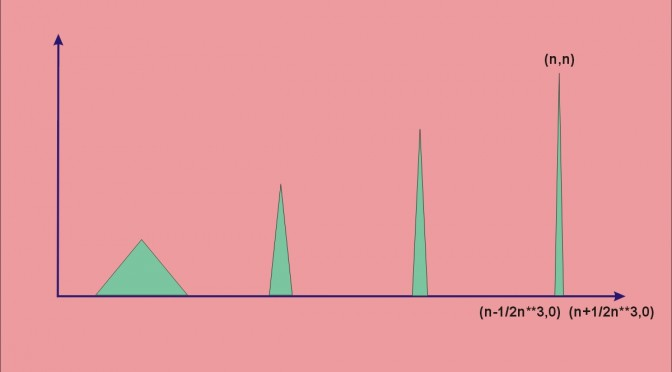# An unbounded positive continuous function with finite integral

Consider the piecewise linear function $$f$$ defined on $$[0,+\infty)$$ taking following values for all $$n \in \mathbb{N^*}$$:
$f(x)= \left\{ \begin{array}{ll} 0 & \mbox{if } x=0\\ 0 & \mbox{if } x=n-\frac{1}{2n^3}\\ n & \mbox{if } x=n\\ 0 & \mbox{if } x=n+\frac{1}{2n^3}\\ \end{array} \right.$

The graph of $$f$$ can be visualized in the featured image of the post.

$$f$$ is positive and continuous, unbounded as $$f(n)=n$$ for all $$n \in \mathbb{N}$$.

We now consider $$\int_0^{+\infty} f$$. For $$X > 0$$, we have:
$\int_0^{X} f \le \int_0^{N + \frac{1}{2N^3}} f$
where $$N=\lceil X \rceil$$ and:
$\int_0^{N + \frac{1}{2N^3}} f = \frac{1}{2}\sum_{n=1}^N \frac{1}{n^2}$
as for all $$n \in \mathbb{N^*}$$:
$\int_{n – \frac{1}{2n^3}}^{n + \frac{1}{2n^3}} f = \frac{1}{2 n^2}$

This proves that the integral of $$f$$ is less than the sum of the convergent series $$(\frac{1}{(n+1)^2})_{n \in \mathbb{N}}$$. Therefore the integral converges.